# Determination of Linear Growth-Rates

Reusing the analysis of Sections 5.75.9, let us again suppose that there are two layers in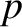space (i.e., Fourier space). The small-layer turns out to be of width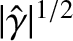, where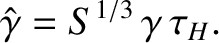(6.4)

Here,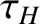is the hydromagnetic timescale defined in Equation (5.43). Given that we are effectively assuming that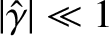, the condition for the separation of the layer solution into two layers (i.e., that the width of the small-layer is less than that of the large-layer) is always satisfied. The large-layer is governed by the equation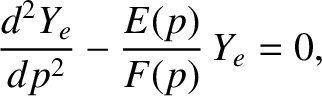(6.5)

where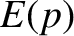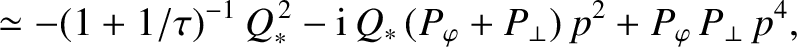(6.6)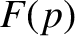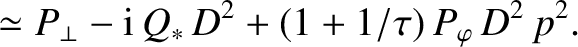(6.7)

Here,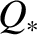is the normalized diamagnetic frequency [see Equation (5.65)],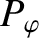and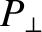are magnetic Prandtl numbers [see Equations (5.53) and (5.54)], and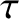is the ratio of the electron to the ion pressure gradient at the rational surface [see Equation (4.5)]. The boundary conditions on Equation (6.5) are that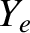is bounded as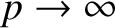, and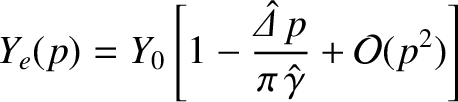(6.8)

as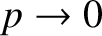. Here,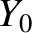is an arbitrary constant.

In the various constant-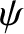linear growth-rate regimes considered in the next section, Equation (6.5) reduces to an equation of the form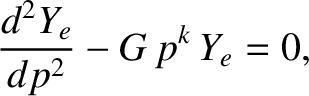(6.9)

where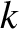is real and non-negative, and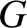is a complex constant. As described in Section 5.8, the solution of this equation that is bounded ascan be matched to the small-asymptotic form (6.8) to give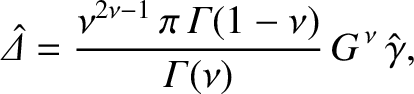(6.10)

where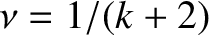. The width of the large-layer inspace is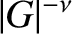.